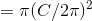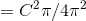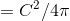High School Math : Circles

Example Questions

Example Question #1 : How To Find Circumference

A circle has the equation below. What is the circumference of the circle?

(x – 2)2 + (y + 3)2 = 9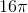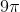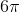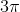Explanation:

The radius is 3. Yielding a circumference of.

Example Question #11 : How To Find Circumference

Ashley has a square room in her apartment that measures 81 square feet. What is the circumference of the largest circular area rug that she can fit in the space?Explanation:

In order to solve this question, first calculate the length of each side of the room.The length of each side of the room is also equal to the length of the diameter of the largest circular rug that can fit in the room. Since, the circumference is simplyExample Question #1 : How To Find The Length Of The Diameter

What is the diameter of a circle with a circumference of?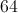Explanation:

To find the diameter we must understand the diameter in terms of circumference. The equation for the circumference of a circle is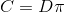, whereis the circumference andis the diameter. The circumference is equal to the diameter multiplied by.

We can rearrangeto solve for.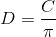All we have to do is plug in the circumference and divide by, and it will yield the diameter.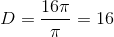Thes cancel and the diameter is.

Example Question #1 : How To Find The Length Of The Diameter

If the area of a circle is four times larger than the circumference of that same circle, what is the diameter of the circle?

2

8

4

16

32

16

Explanation:

Set the area of the circle equal to four times the circumference πr2 = 4(2πr).

Cross out both π symbols and one r on each side leaves you with r = 4(2) so r = 8 and therefore = 16.

Example Question #1 : How To Find The Length Of The Diameter

The perimeter of a circle is 36 π.  What is the diameter of the circle?

3

72

6

18

36

36

Explanation:

The perimeter of a circle = 2 πr = πd

Therefore d = 36

Example Question #1 : Diameter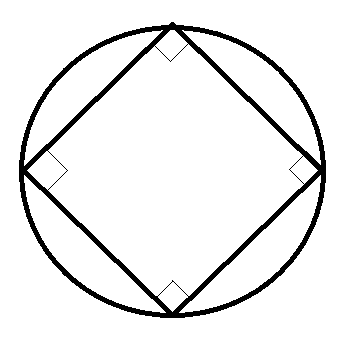If the area of the circle touching the square in the picture above is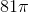, what is the closest value to the area of the square?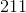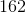Explanation:

Obtain the radius of the circle from the area.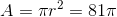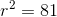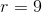Split the square up into 4 triangles by connecting opposite corners. These triangles will have a right angle at the center of the square, formed by two radii of the circle, and two 45-degree angles at the square's corners. Because you have a 45-45-90 triangle, you can calculate the sides of the triangles to be,, and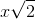. The radii of the circle (from the center to the corners of the square) will be 9. The hypotenuse (side of the square) must be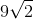.

The area of the square is then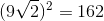.

Example Question #1 : How To Find The Length Of The Diameter

Two legs of a right triangle measure 3 and 4, respectively. What is the area of the circle that circumscribes the triangle?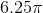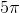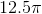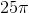Explanation:

For the circle to contain all 3 vertices, the hypotenuse must be the diameter of the circle. The hypotenuse, and therefore the diameter, is 5, since this must be a 3-4-5 right triangle.

The equation for the area of a circle is A = πr2.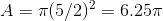Example Question #1 : How To Find The Ratio Of Diameter And Circumference

What is the ratio of the diameter of a circle to the circumference of the same circle?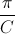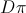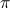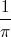Explanation:

To find the ratio we must know the equation for the circumference of a circle. In this equation,is the circumference andis the diameter.Once we know the equation, we can solve for the ratio of the diameter to circumference by solving the equation for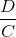. We do this by dividing both sides by.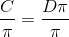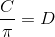Then we divide both sides by the circumference.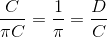We now know that the ratio of the diameter to circumference is equal to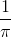.

Example Question #2 : How To Find The Ratio Of Diameter And Circumference

What is the ratio of the diameter and circumference of a circle?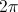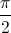Explanation:

To find the ratio we must know the equation for the circumference of a circle is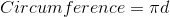Once we know the equation we can solve for the ratio of the diameter to circumference by solving the equation for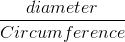we divide both sides by the circumference giving us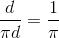We now know that the ratio of the diameter to circumference is equal to.

Example Question #1 : How To Find The Ratio Of Diameter And Circumference

Letrepresent the area of a circle andrepresent its circumference. Which of the following equations expressesin terms of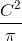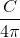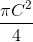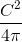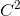Explanation:

The formula for the area of a circle is, and the formula for circumference is. If we solve for C in terms of r, we get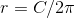.

We can then substitute this value of r into the formula for the area: